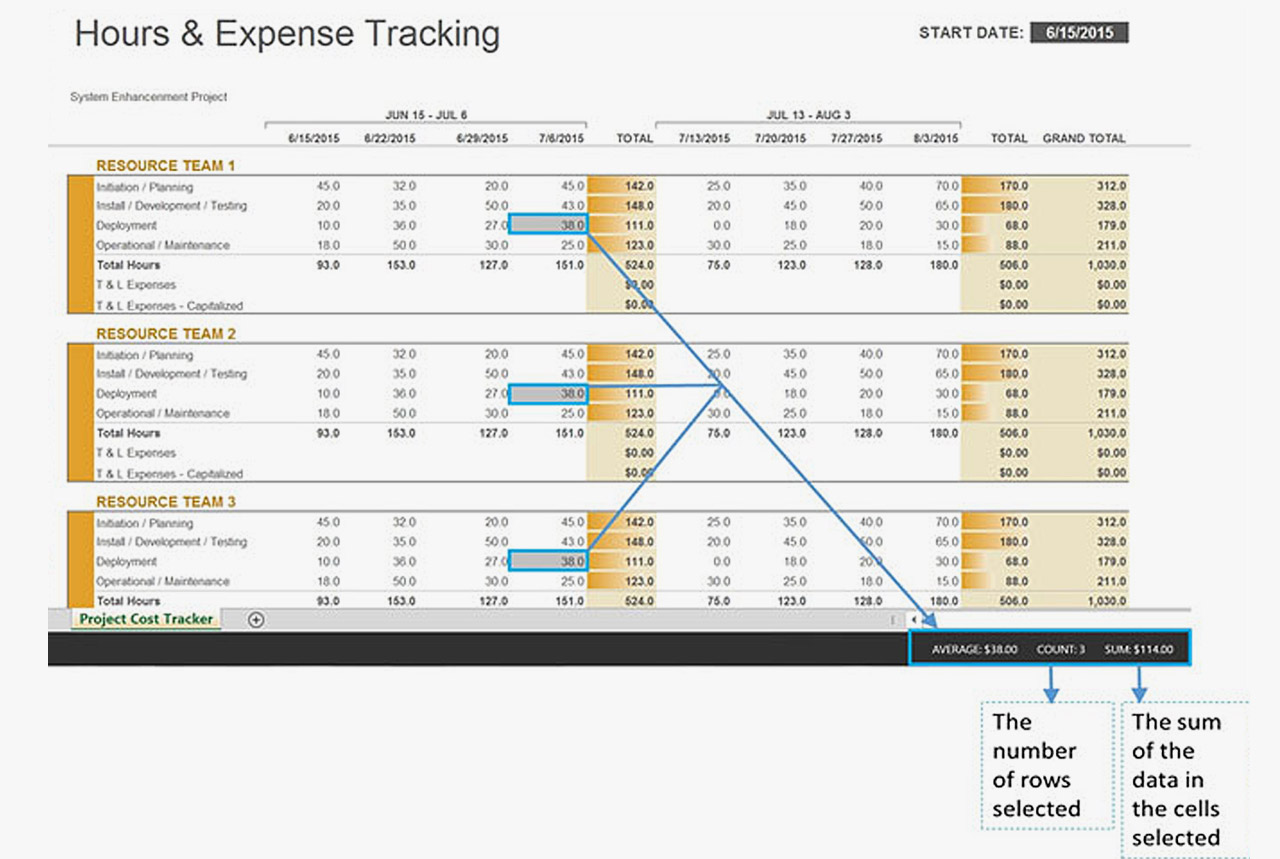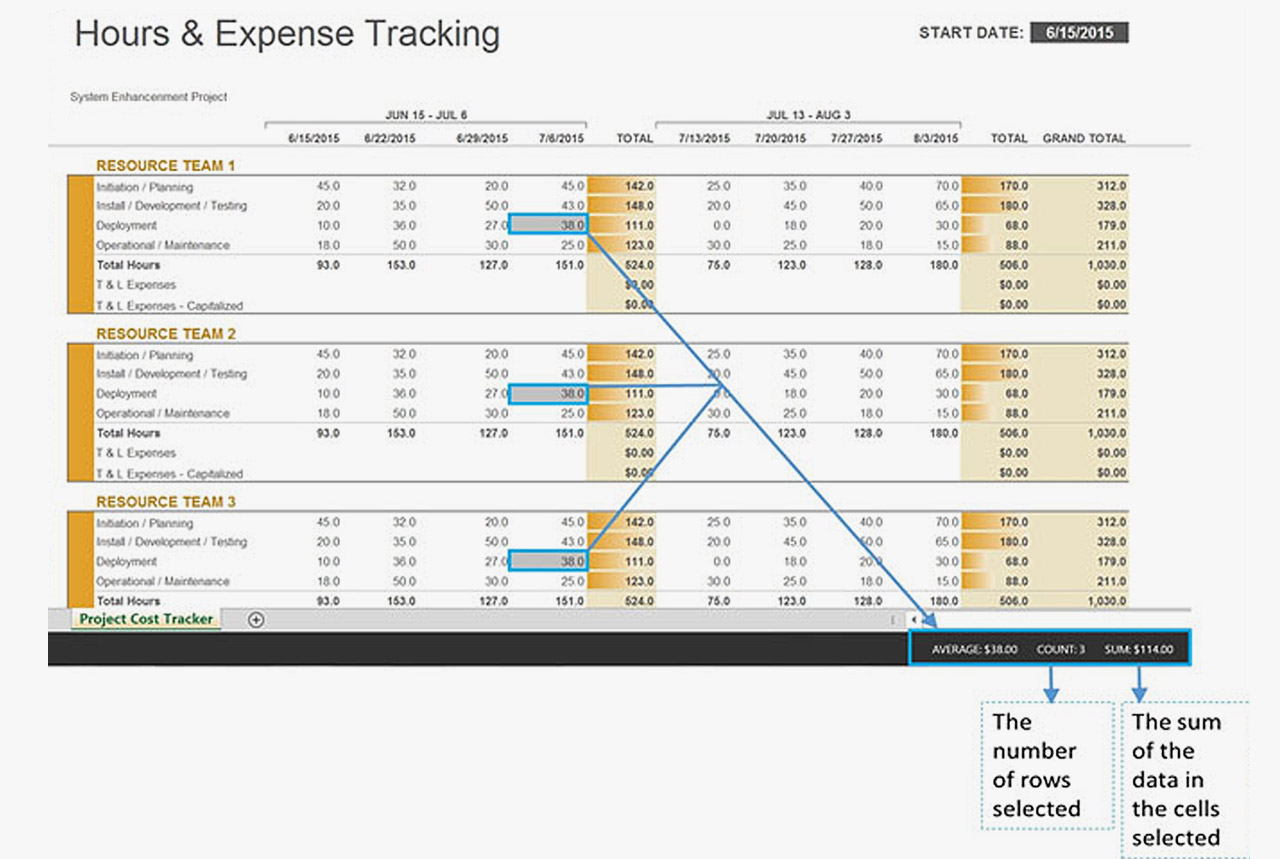# How to Use Microsoft Excel SUM Formula to Manage Data, Minimize Error

As project managers, we often deal with large amounts of data that must be added or counted in a quick and reliable way that also minimizes human errors. Calculating project financials, totaling resource hours, or reconciling large sets of data are all ideal reasons to use a Microsoft Excel SUM formula. While there are numerous ways to calculate formulas, we’re sharing methods that have saved us time:

1. Microsoft Excel SUM Formula (the simple way) – Let’s say you need to quickly add up the total hours spent on a project for a particular resource or a team of resources. This can be done quickly by first selecting the cells of data, and then looking at the information on the bottom right hand corner of your Excel window, as illustrated in the screenshot below. Excel will not only SUM the data, but it will also COUNT how many rows of data are in your selection.

In this screenshot, the project manager wants to know how many hours Team 1 will work on various activities during the week of July 6th. By selecting the cells for that week, she not only sees the total hours for her selection, but also the number of rows selected (COUNT) and the average amount of hours per activity (AVERAGE) – all with a simple cell selection.2. SUM and COUNT (using formulas) – Let’s assume you want the SUM or COUNT of data that reside in non-adjacent rows or columns. You can still use the simple process in the step above, but you’ll first need to select the data cells. You can then apply the SUM formula using one of these methods:

• Using a selection short-cut key – Let’s say that the project manager wants to know the number of hours projected for “Deployment” activities for ALL resource teams the week of July 6th. You can select the cell for your first data point > hold down CTRL and select the next data point > keep your CTRL key held down and select the next data point. Repeat these steps for as many data cells as you want to select, and then release the CTRL key. All selected cells should be highlighted. Now look at the bottom right corner of your Excel window. You should see the AVERAGE, COUNT AND SUM displayed for the data cells you selected.• In some scenarios, you may want to write out a Microsoft Excel SUM formula: =SUM(Number1, Number2, … Number255).While this method requires a few more steps, a formula does make more sense in some instances, like when you’re combining functions.For example, let’s say the project manager wants to know the total number of hours worked on “Deployment” activities divided by the number of days in that week. A formula might be more useful. You would use a SUM to get the total Deployment hours and combine it with a division statement.
In the first example where we wanted the total hours for Team 1 the week of July 6th, the formula would look like this: =SUM(H11:H14). The numbers inside the parenthesis represent the range of cells being added.• In the second example, where data is being added from non-adjacent rows or cells to get the time spent on just “Deployment” activities for all teams, the formula would look like this: =SUM(I13,I21,I29). The numbers inside the parenthesis represent the cell location for each number.For more information on how to use the formula method for SUMS, check out Microsoft’s SUM support page.

Every great project manager should be well versed in Excel, the most widely used spreadsheet in the world today. When you master Excel, you add a powerful tool to your toolkit and ultimately work smarter instead of harder, ten-fold.

Chrystal Richardson is Managing Partner of CE Wilson Consulting, a project management and business efficiency consulting firm that has managed projects for technology, mining, medical, utility and manufacturing clients since 2001. Her PM DNA blog brings together the head and heart of project of management.

#### Chrystal Richardson

PM DNA Blog - by Chrystal Richardson
Project Management